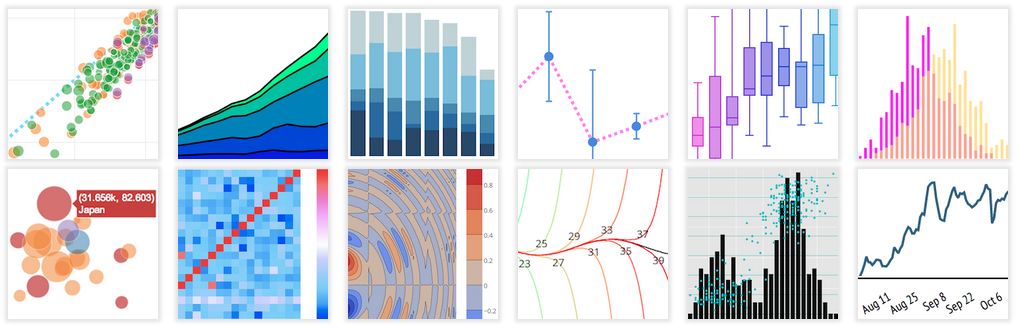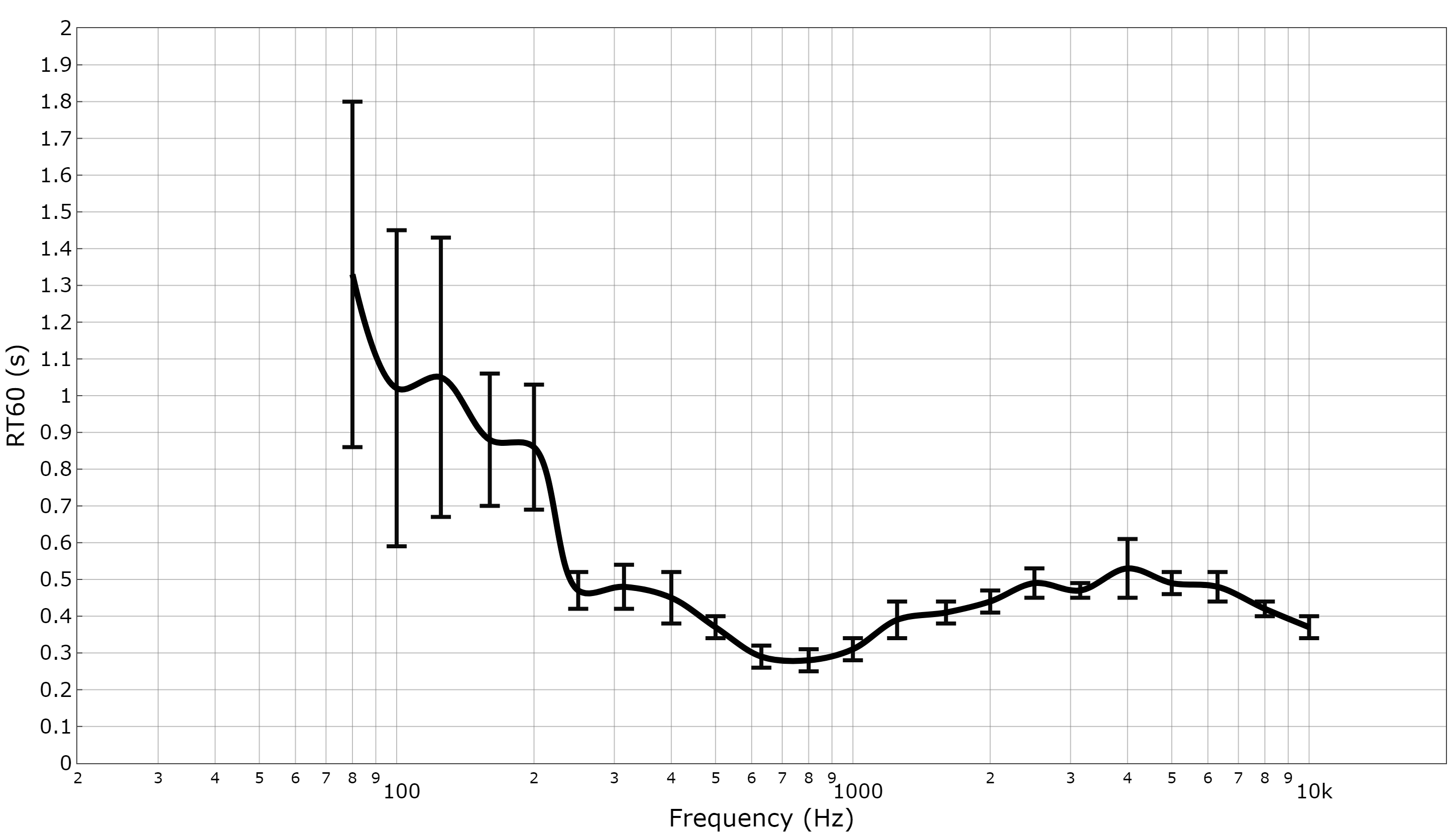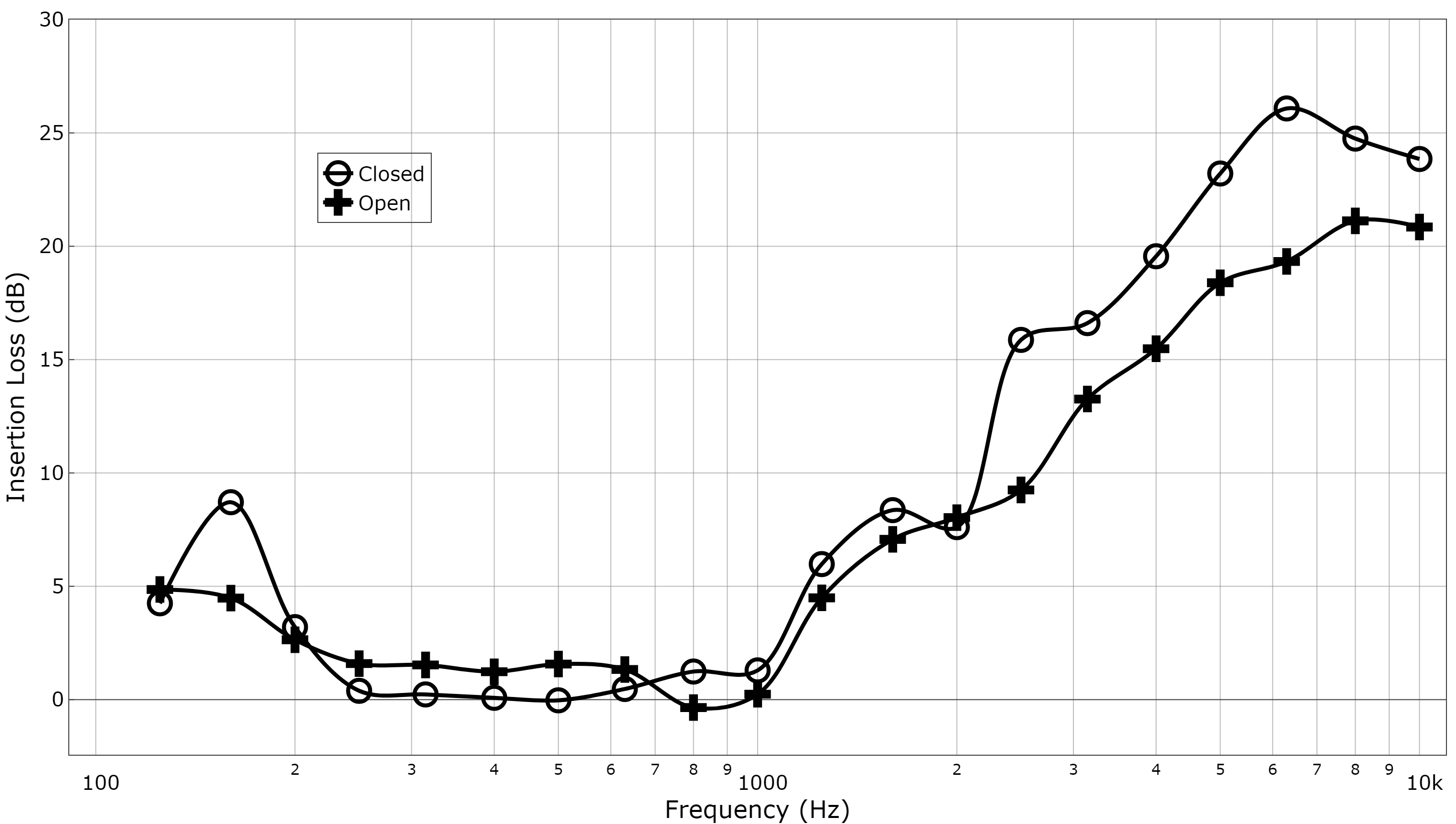# Plotting Audio Data With R & PlotlyDisclaimer: This guide is written for Windows users however all tools are also available for Mac and Linux.

Presenting data in an aesthetically pleasing way can be difficult, there are many different tools available for creating graphs and plots but many of the best are challenging to learn, expensive, or both. This post will show you a way of using the free R programming language along with the graphing service Plotly to create high quality plots, specifically when dealing with audio data.

Plotly is a very powerful web based plotting tool, and for creating one-off plots is very effective, however to use the full range of features you will need to pay a yearly subscription fee of \$99 as a student, or considerably more for a personal account. The other major downside to the online service is the inability to save a template. This makes it very difficult to maintain a particular style across multiple plots. Luckily Plotly is open source and offers API libraries to work in a number of different languages including Python, Matlab, and R.

When working with audio there are a number of different types of data that we are likely to encounter, either in the time domain or the frequency domain. This data is likely stored in a CSV or Excel file, with the first column containing time or frequency information, while subsequent columns contain the data to be plotted. We may also want to plot audio directly from a wav file, either in the time domain or in the frequency domain after FFT processing.

Follow the links below to go to specific plot type or continue reading to see an introductory example.

Below you will find instructions on how to install R, create a script, download the required packages, import some data, create a plot, and export it to an image file.

# Installation

The first thing that we need to do is install R. Next we need to install R Studio, an IDE for R that includes a code editor as well as debugging & visualization tools. Once complete open up R studio.

You should see a console/terminal window, an environment window (environment/history/connections) and a viewer window (files/plots/packages/help/viewer).

You can create a new R script (File>New File) or use an example script. To follow the examples below download:

• RT60 Plot.R
• RT60 Data.txt

and…

• Insertion loss plot.R
• Insertion loss data.txt

From here.

## Packages

First we need to load in a number of packages. To do this we will first create a function to check whether the packages are already installed and then install them if not:

```check.packages <- function(pkg){
new.pkg <- pkg[!(pkg %in% installed.packages()[, "Package"])]
if (length(new.pkg))
install.packages(new.pkg, dependencies = TRUE)
sapply(pkg, require, character.only = TRUE)
}

packages<-c("devtools", "tuneR")
check.packages(packages)```

Next we will use one of our newly installed packages (devtools) to install the latest development version of plotly:

`devtools::install_github("ropensci/plotly")`

Now we need to make sure that the plotly package and the tuneR package are loaded each time we run the script:

```library(plotly)
library(tuneR)```

You may be prompted to install a number of other packages depending on your system, and the installation may take a few minutes (watch the console!). The installation will take place when you first run a script, but first we need to setup the image export function.

### Exporting

In order to export our completed plot we need to use Plotly’s Orca (Open-source Report Creator App) package. Download the relevant installer from here. You will need to add this to your PATH so that it can be called on from R. To do this right-click on the desktop shortcut for Orca and open the properties. Copy the “Start-in” file location. Now go to Control Panel>System and Security>System>Advanced System Settings and under the “Advanced” tab click “Environment Variables…”. Under “System Variables” find “Path” and click “Edit…”, now paste the Orca location into a new line and hit “OK”. To check that this is working correctly open up a cmd prompt and type “orca”. You should see the available commands for the Orca service.

You’re now ready to export a file. Go back to R and add the following line to the end of your project:

`orca(p, "plot.png",scale=2)`

This will export your plot to the working directory (default is My Documents) as a PNG file at double the resolution set earlier.

To see all of the available commands for orca open a cmd prompt and type “orca graph –help”. You can export as PNG, JPEG, webp, svg, pdf, or eps.

Now that we’re setup with all of the necessary packages we can import some data and run a script.

# Using R

End with p to show your plot within RStudio’s viewer, comment out (#) the use of orca until you are happy with your plot in the viewer.

To run your code select all using CTRL + A and run using CTRL + ENTER.

Once you have imported some data using the dialog box you do not need to do it again. The next time you run your script click cancel on the dialog box or comment out that line. You can make quick edits to your plot this way.

You can find documentation relating to R and Plotly here:

• For general tips refer to Plotly’s R cheat sheet found here.
• For examples of different plots see here.
• For detailed information on all the functions of Plotly within R see here.

# Example – Frequency Domain Data from a CSV FileIn this example we will create a plot for 1/3 octave band data, showing one trace with error bars. Our text file is constructed with frequency information in the first column (100, 125, 160, etc.), measurement data (mean RT60) in the second column, and standard deviation in the third column.

We are going to use a dialog box to select our data file, to do this use:

```myFile <- file.choose()

This is going to insert our data into a data frame called “mydata”.

Now we are ready to start using Plotly functions to create our plot.

First we need to create a plot using plot_ly and define the size. Next we need to define our traces and start adding them to the plot. This means selecting the type (line, bar, pie, etc.), shape (straight/curved lines), colour, width, symbol type, etc. There are many different options here, most of which are documented here.

#### EXAMPLE CODE:

```p <- plot_ly(x = mydata[],width=1450,height=900)%>%
y = mydata[],
error_y = list(
array = c(mydata[]),
color = "rgb(10, 10, 10)",
thickness = 2.5,
width = 8.5
),
line = list(
color = "rgb(0, 0, 0)",
shape = "spline",
width = 4
),
mode = "lines",
name = "Closed",
type = "scatter"

)%>%```

In this example trace 1 is made up of plotting column 1 against column 2, which can be chosen using:

x = mydata[],
y = mydata[],

Error bars are added using error_y.

Now we need to define the plot itself, font sizes, colours, axis limits, titles, legends, etc.:

```layout(
autosize = FALSE,
font = list(size = 20, color="rgb(0, 0, 0)"),
hovermode = "closest",
legend = list(
x = 0.18,
y = 0.819,
borderwidth = 1,
traceorder = "normal"
),
margin = list(
t = 100,
),
paper_bgcolor = "rgba(0, 0, 0, 0)",
plot_bgcolor = "rgba(0, 0, 0, 0)",
showlegend = FALSE,
xaxis = list(
autorange = FALSE,
gridcolor = "rgba(127, 127, 127, 0.5)",
gridwidth = 1,
mirror = TRUE,
range = c(0.3, 4.3),
showline = TRUE,
side = "bottom",
title = "Frequency (Hz)",
type = "log"
),
yaxis = list(
autorange = FALSE,
gridcolor = "rgba(127, 127, 127,0.5)",
gridwidth = 1,
mirror = TRUE,
nticks = 13,
range = c(0, 2),
showline = TRUE,
side = "left",
ticks = "inside",
title = "RT60 (s)",
type = "linear"
)
)```

For grid and background colours use rgba to control the transparency of your plots.

When showing more than one trace it may be useful to show a legend, as well as different markers for each trace. The example below shows insertion loss for a window that is either open or closed:The legend is added under layout using:

```legend = list(
x = 0.18,
y = 0.819,
borderwidth = 1,
traceorder = "normal"```

```  marker = list(
color = "rgb(0, 0, 0)",
line = list(
color = "rgb(0, 0, 0)",
width = 0
),
size = 22,
symbol = "cross"
),
mode = "lines+markers",```

You should now have everything you need to create and export a plot. See the list of plot types at the start of this guide if you want to try creating other plots.

### One last tip…

If you’re struggling to get a plot looking how you want you can use Plotly’s web service to help. Create a plot online and use the UI to get it looking how you want, hit save and then view it. At the top of the page you’ll see a heading that says “Python & R”, click that and select R from the drop down menu. Now you’ll be able to see the script behind your plot, copy out the parts you need into your R script and you’re good to go! You’ll notice that the script shown online is constructed a little differently to the examples shown here, so don’t copy everything, just the parts that you need.